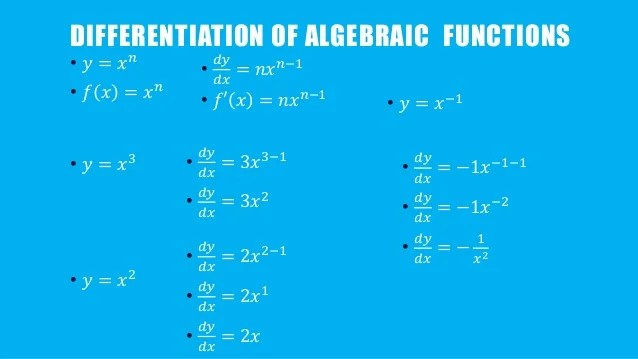# Differentiation of Algebraic Functions II

Welcome to class!

In today’s class, we will be talking more about differentiation of algebraic functions. Enjoy the class!

### Differentiation of algebraic functions II#### Rules of diﬀerentiation

The basic rules of diﬀerentiation are presented here along with several examples. Remember that if y = f(x) is a function then the derivative of y can be represented by dy/dx or y′ or f′ or df /dx.

• ##### Rule 1:

The Derivative of a Constant. The derivative of a constant is zero.

• ##### Rule 2:

The General Power Rule. The derivative of xn is nxn−1.

• ##### Rule 3:

The Derivative of a Constant times a Function.

The derivative of kf (x), where k is a constant, is kf′ (x).

For example:

Diﬀerentiate y = 3x2.

In this case f(x) = x2 and k = 3, therefore the derivative is 3×2x1 = 6x.

• ##### Rule 4:

The Derivative of a Sum or a Diﬀerence.

If f(x) = h(x)±g(x), then df/dx = dh/dx ± dg/dx.

Example:

Diﬀerentiate f(x) = 3x2 −7x.

In this case k(x) = 3x2 and g(x) = 7x and so dk/dx = 6x and dg/dx = 7.

Therefore, df/dx = 6x−7.

• ##### Rule 5: The product rule

The derivative of the product y = u(x) × v(x), where u and v are both functions of x is

dy/dx = u × dv/dx + v × du/dx.

For example:

Diﬀerentiate f(x) = (6x2 + 2x) (x3 + 1).

Let u(x) = 6x2 + 2x and v(x) = x3 + 1.

Therefore, du/dx = 12x + 2 and dv/dx = 3x2.

Using the formula for the product rule, i.e. df /dx = u × dv/dx + v × du/dx

we get,

df/dx = (6x2 + 2x) (3x2) + (x3 + 1) (12x + 2),

= 18x4 + 6x3 + 12x4 + 2x3 + 12x + 2,

= 30x4 + 8x3 + 12x + 2.

• ##### Rule 6: The quotient rule

The derivative of the quotient f(x) = u(x) / v(x), where u and v are both function of x is

df/dx = (v × du/dx – u × dv/dx) / v2.

Example:

Diﬀerentiate f(x) = x2 + 7 / 3x − 1.

Let u(x) = x2 + 7 and v(x) = 3x − 1. Diﬀerentiate these to get du/dx = 2x and dv/dx = 3.

Now using the formula for the quotient rule we get,

df/dx = [(3x−1) (2x) − (x2 + 7) (3)] / (3x−1)2,

= [6x2 − 2x − 3x2 – 21] / (3x−1)2,

df/dx = [3x2 −2x−21] / (3x−1)2.

• ##### Rule 7: The chain rule

If y is a function of u, i.e. y = f(u), and u is a function of x, i.e. u = g(x) then the derivative of y with respect to x is

dy/dx =dy/du × du/dx.

Example:

Diﬀerentiate y = (x2 −5)4.

Let u = x2 − 5, therefore y = u4.

du/dx = 2x             and           ⇒ dy/du = 4u3.

Using the chain rule, we then get

dy/dx = dy/du × du/dx,

= 4u3 ×2x,

= 4(x2 −5)3 ×2x,

= 8x (x2 −5)3.

In our next class, we will be talking about Integration and Evaluation of Simple Algebraic Functions. We hope you enjoyed the class.

Should you have any further question, feel free to ask in the comment section below and trust us to respond as soon as possible.

For more class notes, videos, homework help etc download our Mobile App HERE

Join ClassNotes.ng Telegram Community HERE for exclusive content and support

Don`t copy text!